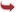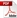International Journal of Scientific and Research Publications

#### IJSRP, Volume 4, Issue 8, August 2014 Edition [ISSN 2250-3153]Degree Based Topological Indices of Isomers of Organic Compounds
Dr N K Raut
Abstract: Let G(V,E) be a connected graph. The sets of vertices and edges of G are denoted by V=V (G) and E=E (G) respectively. In such a molecular graph, vertices represent atoms and edges represent bonds. The number of edges incident on a vi is called the degree d(vi) of vertex vi.The sum of degrees of all vertices in G is twice the number of edges in G . In molecular graph we have many topological indices. In this research, we computing Randic index, Molecular topological index ,Augmented Zagreb index ,Geometric-Arithmetic index ,Atom–bond connectivity index, Harmonic index ,Sum-connectivity index of n-decane ,3,4,4-Trimethyl heptane and 2,4-dimethyl-4-ethyl hexane.
[VIEW FULL PAPER][DOWNLOAD]

Reference this Research Paper (copy & paste below code):

Dr N K Raut (2018); Degree Based Topological Indices of Isomers of Organic Compounds ; Int J Sci Res Publ 4(8) (ISSN: 2250-3153). http://www.ijsrp.org/research-paper-0814.php?rp=P323027